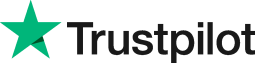3d formulas in Excel 2010
• Home
• Courses
• Promotions
• Schedule
• Formats
• Our Clients

Forum home » Topics » 3d formulas in Excel 2010

# 3d formulas in Excel 2010

The term 3D formulas is used when referring to the same cell on more than one sheet. For example, if you wanted to add together what's in cell B4 on three different sheets and display the result in a fourth sheet.

To create the 3D Formula

On the fourth sheet, type =sum(

Click on the sheet tab for the first sheet, hold down Shift and select the tab for the third sheet, then click cell B4 and press Enter.

The 3D formula created in Sheet4 would be:
=SUM(Sheet1:Sheet3!B4)

The benefits of using a 3D formula in a work setting is when there are many sheets with figures to total up. For example consider the formula
= SUM(Week1:Week52!B4)
This 3D formula totals up a year full of weekly sheets. It would be very time consuming or impossible to do the calculation by adding each individual sheet because of the length of formula required:
=Sheet1!B4+Sheet2!B4+Sheet3!B4 .... etc

The disadvantage of using a 3D formula is that the cells to be added all have to be the same one.

See below to see other questoins about 3D formulas.

# Related forum posts:

## 3-D formulas

I went on the intermediate Excel course and we discussed 3-D formulas. I am trying to do the exercises again but I am getting stuck. My main question is how to create a 3-D formula? I am using the 2003 version of Excel and I can't see the &quot;save workspace&quot; option. I also have read only files and am wondering whether this is restricitng me from creating the formula. I am looking forward to your feedback. Regards Adriaan

## freeze panes.3-D formulas

How do you create 3-D formulas to link worksheets and freeze panes.

## 3D formulas - error tracing

How are errors in 3D formulas traced?

## Excel

Can I use the Average function in a 3d formula? I get error messages.

## 3-D Formulas

How does a watch window help with 3D formulas?

## Working w multiple worksheet

how to create 3-Dformula to link worksheets

## 3D Formulas

How do I do these?

## creating 3d formulas